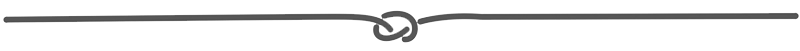## 18 Dec 2014

### A useful tip to convert between miles and kilometres!

Hi there!

Today I'm going to give you a very useful tip to convert between kilometres and miles! It is specially useful for those who like languages but also have a certain mind for Maths! But even if you don't like Maths, read through the end and see how easy it is!

Have you ever heard of the Fibonacci Sequence? I'm sure you have! For those who don't know, the sequence is as follows:

1, 1, 2, 3, 5, 8, 13, 21, 34, 55, 89, 144...

How does it work? Well, each number results from adding up the two neighbouring numbers:

Now, one interesting aspect of these numbers is that their ratios (divisiones) all approach being what is called the golden ratio: 1,618. So, for example,

• 3 divided by 2 is 1,5;
• 5 divided by 3 is 1,666;
• 8 divided by 5 is 1,6;
• 13 divided by 8 is 1,625;
• 21 divided by 13 is 1,615, etc.

And... what does this have to do with kilometres and miles? Well, curiously enough, the ratio of km to miles is 1,609 to 1. Since 1,609 is so close to the golden ratio, you can use the Fibonacci numbers to convert between kilometres and miles!!

• 5 km is quite close to 3 miles
• 8 km is almost exactly 5 miles
• 13 km is 8 miles
• 21 km is 13 miles, etc.!

Now you won't have any problem with distances when you travel to the United Kingdom! :)